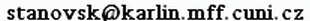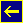David Stanovský    //UNIVERSAL ALGEBRA I (2021/22)

This is an introductory course in universal algebra, essentially following Bergman's textbook. We will learn a general framework for elementary algebraic concepts such as factoring or direct decomposition. We will introduce free algebraic structures and learn the basics of equational logic. We will present the modern approach using clones, i.e., sets of operations closed with respect to composition.

We will mostly follow a textbook by Clifford Bergman, Universal Algebra: Fundamentals and Selected Topics.

Syllabus:

1. Preliminaries - algebras, lattices, closure operators [1.1-1.2, 2.1, 2.3-2.5]
2. Semantics - subalgebras, products, homomorphisms, quotients, isomorphism theorems, internal/external characterisation of products, subdirect irreducibility [1.3-1.5, 3.1-3.5]
3. Syntax - terms, free algebras, equational classes, Birkhoff's theorem, fully invariant congruences [4.2-4.6]
4. Clones - interpretations, algebraic vs. relational clones [4.1, 4.8 and other notes]
5. Classification schemes - Maltsev conditions, abelianness [4.7 and other notes]

You can look at the 18/19 version, this year will be similar.

Program (actual for past lectures, tentative for future lectures):

 covered topics recommended reading homework 4.10. Motivation. Examples of algebras and equational classes. Ex.: Isomorphism. Lattices as ordered sets and as algebras. Bergman 1.1, 1.2 11.10. Introduction to lattices, complete lattices, closure operators. Ex.: The lattice of equivalence relations. Modularity and distributivity. Closure operators. Bergman 2.1, 2.2, 2.3 HOMEWORKdue on 26.10. 18.10. Algebraic lattices, algebraic closure operators. Galois correspondences. Ex.: Algebraic closure operators. Galois correspondences. Bergman 2.4, 2.5 25.10. Subalgebras, subalgebra generation. Congruences and quotients. Ex.: Calculating Sg, Sub, homomorphisms. Bergman 1.3-1.5 1.11. Congruence generation. Homomorphic images and isomorphism theorems. Ex.: Calculating Cg, Con, quotients. Bergman 1.3, 3.1 HOMEWORKdue on 16.11. 8.11. Direct product and subpowers. Direct decomposition. H,S,P operators and varieties (part 1). Ex.: Direct decomposition. Bergman 3.2, 3.5 15.11. H,S,P operators and varieties (part 2). Subdirect decomposition. Ex.: Subdirect decomposition. Bergman 3.5, 3.3 22.11. Examples of subdirectly irreducible algebras. Terms, identities and absolutely free algebras. Ex.: Subdirect decomposition. Free algebras. Bergman 4.3 HOMEWORKdue on 7.12. 29.11. Free algebras in particular varieties. The syntax-semantics Galois connection and Birkhoff's theorem. Ex.: Free algebras. Bergman 4.4 6.12. Equational theories as fully invariant congruences. Functional clones. Ex.: Clones of term and polynomial operations. Bergman 4.6, 4.1 HOMEWORKdue on 21.12. 13.12. Free algebras as clones of term functions. The Pol-Inv connection (between functions and relations). Ex.: Pol, Inv, generating clones. Bergman 4.2 (covers only partly) look at Post's lattice 20.12. Relational clones and the Pol-Inv connection. Ex.: clones and relational clones. Bergman 4.7 Pol-Inv notes HOMEWORKdue on 4.1. 3.1. Maltsev conditions. Ex.: Maltsev conditions.

Literature:

Zapocet: There will be five series of homeworks, the four best count. Of the four best, you must have at least 50% in each series and 60% together.

Exam: preliminary format will be as follows (to be adjusted after the first exam)

1. (40-50%) quizz (statement of definitions, theorems, simple examples, simple proofs) - collected and graded after 60 minutes
2. (50-60%) 2-3 serious questions (difficult proof, 1-2 problems) - oral presentation whenever ready
Summary of topics and typical problems is here.